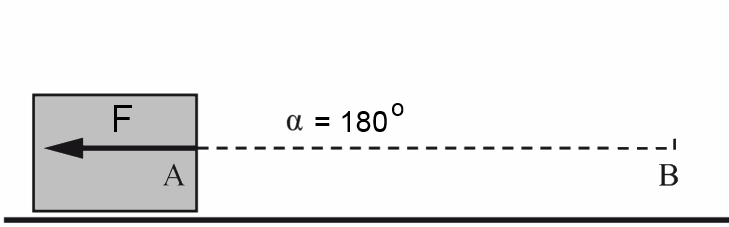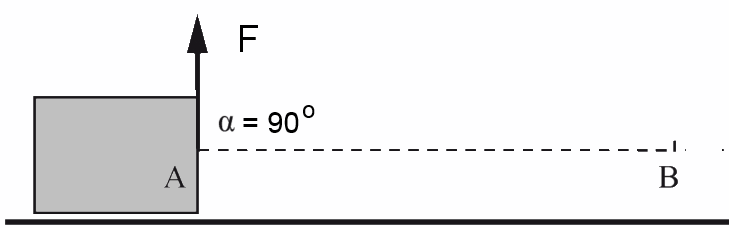Search:

# Work

## Definition

A body moves from A to B driven by a force F which makes an angle α with its trajectory. The force F performs a work $W$$W$ = (projection of $F$ on $AB$) · $AB$ $W$= $F'$ · $AB$

$W$ $=$ $F \cdot AB \;cos \alpha$

## Unit

1 Newton · 1 meter = 1 Joule $1 J$ $=$ $1 N\;m$

## Special cases$W$ $=$ $F\cdot AB \cdot cos0^o$

$W$ $=$ $F\cdot AB$$W$ $=$ $F\cdot AB \cdot cos180^o$

$W$ $=$ $-F\cdot AB$$W$ $=$ $F\cdot AB \cdot cos90^o$

$W$ $=$ $0$

## ExampleA skier weighing $650\; N$ races down the slope of a mountain on a trail of $2000\;m$ which makes an angle of $60^o$ with the vertical. Calculate the work done by his weight. $W_P$ $=$ $650 \cdot 2000 \cdot cos 60^o$ $=$ $650000 \;J$Covering a distance of $2000\;m$, a skier weighing $650\;N$ uses a ski lift which makes an angle of $110^o$ with the vertical. Calculate the work done by his weight. $W_P$ $=$ $650 \cdot 2000 \cdot cos 110^o$ $=$ $-444626 \;J$